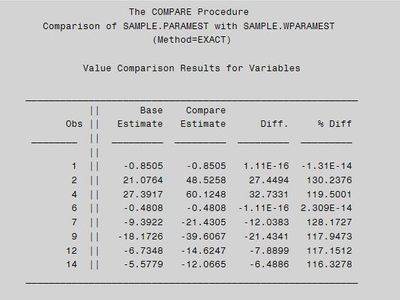## Proc logistic with class variable - weighted vs. unweighted

Dear All,

I came across a problem when I ran proc logistic with class variable with weights and without weights. For some cases I got "close" the same estimates while for others totally diferrent.

The input data set (SAMPLE.zip - 60 rows) is attachted to this post as well as the SAS code I executed.

``````proc sort data=sample.sample;
by class_var var1 var2;
run;

/*Generate weights*/
proc summary data=sample.sample nway;
class class_var var1 var2 target_var;
output out=sample.weights(drop=_type_ rename=(_freq_= weight));
run;
/*With weights*/
proc logistic data = sample.weights;
class class_var /param = GLM;
model target_var(EVENT = '1') = class_var  var1 * class_var  var2 * class_var /noint;
weight weight;
ods output ParameterEstimates = sample.wparamest Association = sample.wassocest;
run;
title;
/*Without weights*/
proc logistic data = sample.sample;
class class_var /param = GLM;
model target_var(EVENT = '1') = class_var  var1 * class_var  var2 * class_var /noint;
ods output ParameterEstimates = sample.paramest Association = sample.assocest;
run;
title;

proc compare base=sample.paramest compare=sample.wparamest;
run;``````

There are two cases:

1. Using the sample data set without weights (in this case the input table has 60 rows)

2. Using the weights table containing the weight variable that is also used in the proc logistic. (in this case the input table has 57 rows, in 3 cases the weights are 2).

When I compare the results I get differencies as follows:Could you please tell me what could cause these differencies?

Thank you!

BR,

Gabor

1 ACCEPTED SOLUTION

Accepted Solutions

## Re: Proc logistic with class variable - weighted vs. unweighted

You are confusing the WEIGHT statement with the FREQ statement.  If you use a FREQ statement in the first PROC LOGISTIC call, the values agree to within about 1e-15, which is what you would expect. For details, see "The difference between frequencies and weights in regression analysis".

4 REPLIES 4

## Re: Proc logistic with class variable - weighted vs. unweighted

You are confusing the WEIGHT statement with the FREQ statement.  If you use a FREQ statement in the first PROC LOGISTIC call, the values agree to within about 1e-15, which is what you would expect. For details, see "The difference between frequencies and weights in regression analysis".

## Re: Proc logistic with class variable - weighted vs. unweighted

Thank you Rick for your comment, using FREQ instead of WEIGHT solved my problem.

There is still one thing that is not clear for me. In the article you say the followings:

1. "A frequency variable tells the procedure that there are more observations than there are rows in the data set. When you run a frequency analysis, your analysis should agree with the same analysis run on the "expanded data," which is the data set in which each row represents a single observation."
2. "In the regression context, if you use integer counts as weights, the parameter estimates are the same as when you use the counts for frequencies".

From 1 and 2 I have the parameter estimates with integer weights = parameter estimates with frequencies = parameter estimates for the "expanded data".

However, in my test case this clearly does NOT hold since some of my parameter estimates are different.

What could be the issue here?

## Re: Proc logistic with class variable - weighted vs. unweighted

I wrote that article for LINEAR regression. As you have observed, the weights affect the parameter estimates nonlinearly in logisitc regression and other generalized regression models.

## Re: Proc logistic with class variable - weighted vs. unweighted

Thank you, now it is clear.

Discussion stats
• 4 replies
• 2917 views
• 0 likes
• 2 in conversation